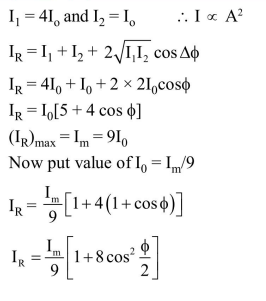Deepak Scored 45->99%ile with Bounce Back Crack Course. You can do it too!

# In Young's double slit experiment, one of the slit is wider than other,

Question:

In Young's double slit experiment, one of the slit is wider than other, so that the amplitude of the light from one slit is double of that from other slit. If $\mathrm{I}_{\mathrm{m}}$ be the maximum intensity, the resultant intensity I when they interfere at phase difference $\phi$ is given by :

1. $\frac{I_{m}}{9}\left(1+8 \cos ^{2} \frac{\phi}{2}\right)$

2. $\frac{\mathrm{I}_{\mathrm{m}}}{9}(4+5 \cos \phi)$

3. $\frac{\mathrm{I}_{\mathrm{m}}}{3}\left(1+2 \cos ^{2} \frac{\phi}{2}\right)$

4. $\frac{\mathrm{I}_{\mathrm{m}}}{5}\left(1+4 \cos ^{2} \frac{\phi}{2}\right)$

Correct Option: 1

Solution: